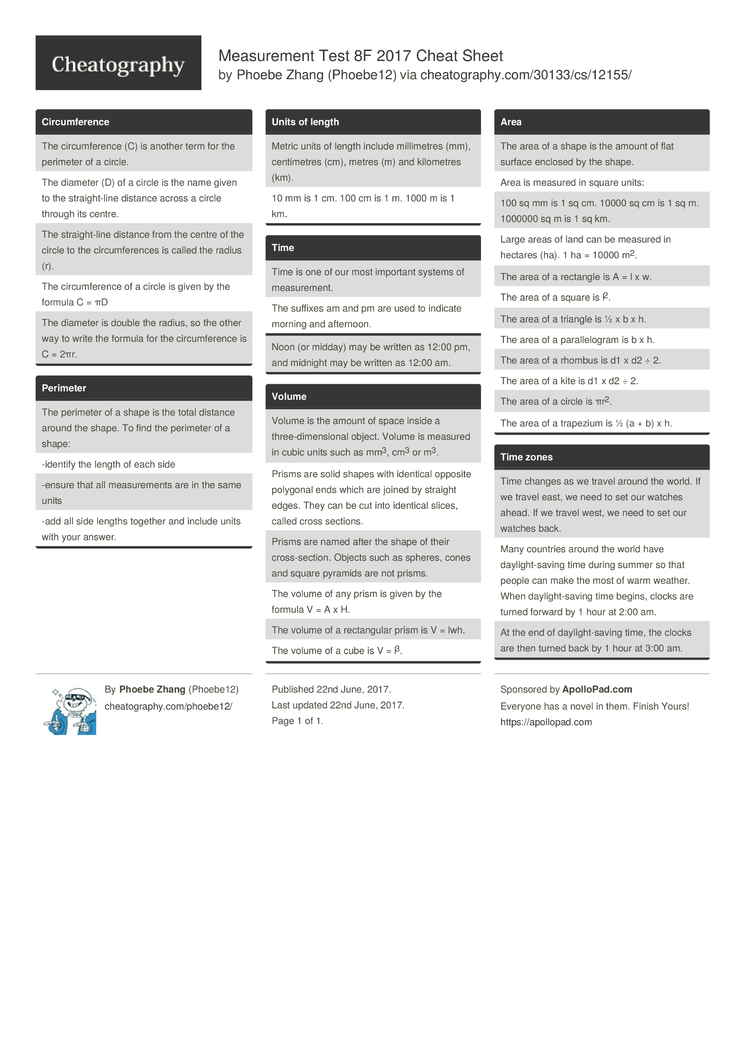Measurement Test 8F 2017 Cheat Sheet by Phoebe12

Circum­ference

 The circum­ference (C) is another term for the perimeter of a circle. The diameter (D) of a circle is the name given to the straig­ht-line distance across a circle through its centre. The straig­ht-line distance from the centre of the circle to the circum­fer­ences is called the radius (r). The circum­ference of a circle is given by the formula C = πD The diameter is double the radius, so the other way to write the formula for the circum­ference is C = 2πr.

Perimeter

 The perimeter of a shape is the total distance around the shape. To find the perimeter of a shape: -identify the length of each side -ensure that all measur­ements are in the same units -add all side lengths together and include units with your answer.

Units of length

 Metric units of length include millim­etres (mm), centim­etres (cm), metres (m) and kilometres (km). 10 mm is 1 cm. 100 cm is 1 m. 1000 m is 1 km.

Time

 Time is one of our most important systems of measur­ement. The suffixes am and pm are used to indicate morning and afternoon.
Noon (or midday) may be written as 12:00 pm, and midnight may be written as 12:00 am.

Volume

 Volume is the amount of space inside a three-­dim­ens­ional object. Volume is measured in cubic units such as mm3, cm3 or m3. Prisms are solid shapes with identical opposite polygonal ends which are joined by straight edges. They can be cut into identical slices, called cross sections. Prisms are named after the shape of their cross-­sec­tion. Objects such as spheres, cones and square pyramids are not prisms. The volume of any prism is given by the formula V = A x H. The volume of a rectan­gular prism is V = lwh. The volume of a cube is V = l3.

Area

 The area of a shape is the amount of flat surface enclosed by the shape. Area is measured in square units: 100 sq mm is 1 sq cm. 10000 sq cm is 1 sq m. 1000000 sq m is 1 sq km. Large areas of land can be measured in hectares (ha). 1 ha = 10000 m2. The area of a rectangle is A = l x w. The area of a square is l2. The area of a triangle is ½ x b x h. The area of a parall­elogram is b x h. The area of a rhombus is d1 x d2 ÷ 2. The area of a kite is d1 x d2 ÷ 2. The area of a circle is πr2. The area of a trapezium is ½ (a + b) x h.

Time zones

 Time changes as we travel around the world. If we travel east, we need to set our watches ahead. If we travel west, we need to set our watches back. Many countries around the world have daylig­ht-­saving time during summer so that people can make the most of warm weather. When daylig­ht-­saving time begins, clocks are turned forward by 1 hour at 2:00 am. At the end of daylig­ht-­saving time, the clocks are then turned back by 1 hour at 3:00 am.

Download the Measurement Test 8F 2017 Cheat Sheet1 Page
//media.cheatography.com/storage/thumb/phoebe12_measurement-test-8f-2017.750.jpg

PDF (recommended)